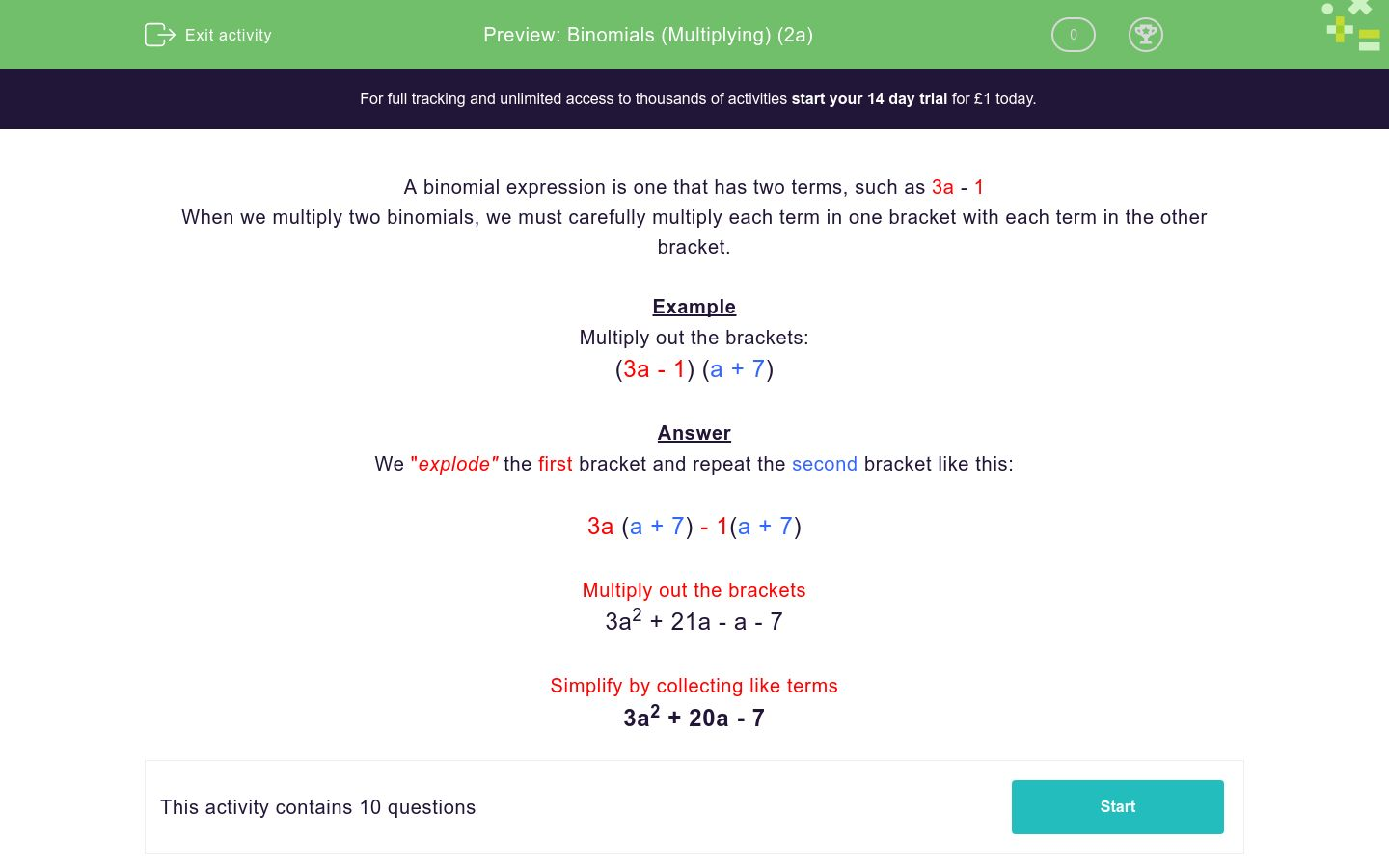# Binomials (Multiplying) (2a)

In this worksheet, students multiply together two binomial expressions.Key stage:  KS 4

Curriculum topic:  Algebra

Difficulty level:### QUESTION 1 of 10

A binomial expression is one that has two terms, such as 3a - 1

When we multiply two binomials, we must carefully multiply each term in one bracket with each term in the other bracket.

Example

Multiply out the brackets:

(3a - 1) (a + 7)

We "explode" the first bracket and repeat the second bracket like this:

3a (a + 7) - 1(a + 7)

Multiply out the brackets

3a2 + 21a - a - 7

Simplify by collecting like terms

3a2 + 20a - 7

Multiply out the brackets:

(3a + 2) (a + 1)

a2+3a +2

3a2+3a +1

3a2+5a +2

a2+2a +1

Multiply out the brackets:

(2a + 2) (a + 3)

2a2+6a +5

a2+8a +6

2a2+8a +6

a2+2a +6

Multiply out the brackets:

(2a + 6) (a + 1)

2a2+8a +6

2a2+6a +7

2a2+8a +5

a2+7a +6

Multiply out the brackets:

(3a + 4) (a - 1)

3a2+4a +3

4a2+a -4

3a2+3a -4

3a2+a -4

Multiply out the brackets:

(a + 3) (3a - 2)

3a2+7a +6

3a2+5a -6

a2+a -6

3a2+7a -6

Multiply out the brackets:

(a + 1) (5a - 6)

4a2+5a -6

5a2-a -6

5a2+11a -6

a2-a -6

Multiply out the brackets:

(5a + 2) (a - 4)

4a2+18a -6

5a2+18a -8

5a2+2a -8

5a2-18a -8

Multiply out the brackets:

(3a + 4) (a - 4)

3a2-16

3a2-8a -16

3a2-4a -8

3a2+8a -16

Multiply out the brackets:

(a - 4) (3a - 4)

3a2-16a +16

3a2-16a -16

3a2+8a -16

3a2-16a -8

Multiply out the brackets:

(4a - 3) (a - 4)

4a2-7a -7

4a2-19a +7

4a2-19a +12

4a2+19a -12

• Question 1

Multiply out the brackets:

(3a + 2) (a + 1)

3a2+5a +2
• Question 2

Multiply out the brackets:

(2a + 2) (a + 3)

2a2+8a +6
• Question 3

Multiply out the brackets:

(2a + 6) (a + 1)

2a2+8a +6
• Question 4

Multiply out the brackets:

(3a + 4) (a - 1)

3a2+a -4
• Question 5

Multiply out the brackets:

(a + 3) (3a - 2)

3a2+7a -6
• Question 6

Multiply out the brackets:

(a + 1) (5a - 6)

5a2-a -6
• Question 7

Multiply out the brackets:

(5a + 2) (a - 4)

5a2-18a -8
• Question 8

Multiply out the brackets:

(3a + 4) (a - 4)

3a2-8a -16
• Question 9

Multiply out the brackets:

(a - 4) (3a - 4)

3a2-16a +16
• Question 10

Multiply out the brackets:

(4a - 3) (a - 4)